# Test: Turbulent Flow in Pipes

## 9 Questions MCQ Test Topicwise Question Bank for Civil Engineering | Test: Turbulent Flow in Pipes

Description
Attempt Test: Turbulent Flow in Pipes | 9 questions in 30 minutes | Mock test for Civil Engineering (CE) preparation | Free important questions MCQ to study Topicwise Question Bank for Civil Engineering for Civil Engineering (CE) Exam | Download free PDF with solutions
QUESTION: 1

Solution:
QUESTION: 2

### In a turbulent flow in a pipe, the shear stress is

Solution:

In turbulent flow shear stress
τ = τ0 (1 - y/r0
where,
y = Distance measured from pipe wall
ζ0 = Shear stress at boundary

QUESTION: 3

### In the case of turbulent flows in circular pipes, the maximum velocity um and the mean velocity V are related as (um - V)lu =

Solution:

Turbulent flow
For smooth pipe,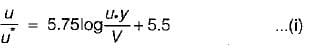Average velocity,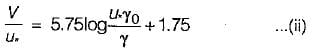y = distance measured from pipe boundary
u = shear velocity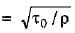from equation (i)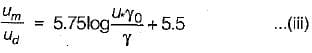(iii) - (ii) gives
(b) Rough pipe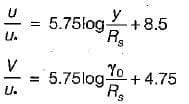It gives,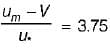same for both pipes rough or smooth

QUESTION: 4

The distance from pipe boundary, at which the turbulent shear stress is one-third the wall shear stress, is

Solution:

In turbulent flow shear stress at distance y from pipe boundary,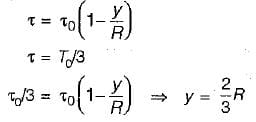QUESTION: 5

A flow in which the quantity of liquid flowing per second is not constant, is called

Solution:

A fluid motion is said to be turbulent when the fluid particles move in an entirely haphazard or disorderly manner, that results in a rapid and continuous mixing of the fluid leading to momentum transfer as flow occurs. At any point in a turbulent flow the velocity and pressure are the functions of time thereby rendering such a flow as unsteady.

QUESTION: 6

Which of following conditions would entail a greater energy dissipation in turbulent flow?
1. Smaller eddy size
2. Lower viscosity
3. Large intensity of turbulence

Select the correct answer using the codes given below:

Solution:

" A large Reynolds number indicates that viscous forces are not important at large scales of the flow. With a strong predominance of inertial forces over viscous forces, the largest scales of fluid motion are undamped—there is not enough viscosity to dissipate their motions. The kinetic energy must "cascade" from these large scales to progressively smaller scales until a level is reached for which the scale is small enough for viscosity to become important (that is, viscous forces become of the order of inertial ones). It is at these small scales where the dissipation of energy by viscous action finally takes place. The Reynolds number indicates at what scale this viscous dissipation occurs.

QUESTION: 7

Match List-I (Nature of flow) with List-II (Friction factor equation) and select the correct answer using the codes given below the lists:
List- I
1.Turbulent flow with rough boundary
2. Turbulent flow with smooth boundary
3. Turbulent flow with Re < 105
4. Laminar flow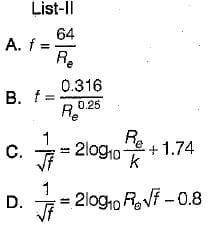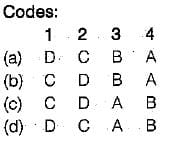Solution:
QUESTION: 8

Turbulent flow generally occurs

Solution:

Reynolds number, Re = Vd/v
When Re < Rec Laminar flow occurs at very small velocities or through narrow passage (small d) or for highly viscous fluids (high v) the Re will be small and flow will remain laminar. For high velocities through large passage Reynolds number will be sufficient to cause turbulence.

QUESTION: 9

A pipe line carrying water has surface-protrusions of average height 0.10 mm. If the shear stress developed is 7.85 N/m2, then the pipe surface will act as

Solution:

ks = 0.10 mm
τ = 7.85 N/m2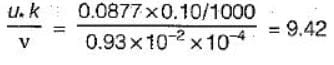Since roughness value lies between 4 and 100 the pipe act as in transition.Use Code STAYHOME200 and get INR 200 additional OFF Use Coupon Code